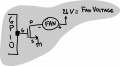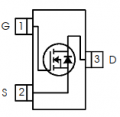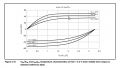# Mosfet as a Switch application

#### kalemaxon89

Joined Oct 12, 2022
61
I have the output of a GPIO of an ST microcontroller which switches high (3.3V) or low (0V).
I want a fan to turn on when the output is high and turn off when it is low.
So I need to apply a simple mosfet (between the gpio and the fan) that switches between the saturation region and the cut-off region (like a switch)

Assuming I use this fan and this mosfet (I know it's not the best choice, but let's assume we're using this), I have some doubts on circuit dimensioning:

1) The chosen mosfet has Vgs(th) = 1.7V ... so it will conduct and turn on the fan if Vgs > 1.7V
Since I have 0V or 3.3V output from the GPIO, can I connect the mosfet directly to the pin? Do I need a resistor or some protection diodes?
Since no current is flowing on the gate, I think the only condition to be met is that any voltage on the input of the pin (i.e. Vgs(th)) does not exceed the maximum breakdown voltage of the pin itself ... right?
If it is true, in this case I don't think it will happen since Vgs(th) << Vgpio tolerant (it should be 3V or 5V)

2) The fan datasheet states that the maximum rated current is 74mA ... this means that my drain current must not exceed that value, is this correct?
But it is not clear to me how I can calculate the drain current flowing when the mosfet conducts in saturation (switch circuit closed).
Once I understand what I have to calculate, I can also definitely add a resistor.#### Ian0

Joined Aug 7, 2020
6,715
So far so good.
The drain current is the operating current of the fan.
The fan is effectively connected to the 24V supply through a 0.027Ω resistor.

#### Papabravo

Joined Feb 24, 2006
19,612
Note of Caution. Vgs(th) is the voltage at which a MOSFET begins to conduct. The voltage you need to achieve for the device to be fully ON may be higher. Make it a habit when looking at MOSFET datasheets to make sure you extract the magnitude of this higher voltage.

Also a GPIO pin is a really terrible gate driver, because you are using it to charge and discharge a big capacitor represented by the MOSFET gate.. Whever you switch the gate you must endeavor to do it rapidly of the the MOSFET will spend excessive time in the linear region acting like a heater and dissipating more power than you might expect. It is possible that 3.3V will put the MOSFET smack dab in the middle of the linear range and you need Vgs = 10V to turn it on fully. Just look at the datasheet to make sure what you are working with.

•#### LowQCab

Joined Nov 6, 2012
2,650
•KeithWalker and kalemaxon89

#### michael8

Joined Jan 11, 2015
333
The chosen mosfet has Vgs(th) = 1.7V ... so it will conduct and turn on the fan if Vgs > 1.7V

No, the mosfet datasheet says at Vgs it will conduct 25 uA not the 74 mA the fan needs.

Since I have 0V or 3.3V output from the GPIO, can I connect the mosfet directly to the pin? Do I need a resistor or some protection diodes? Since no current is flowing on the gate, I think the only condition to be met is that any voltage on the input of the pin (i.e. Vgs(th)) does not exceed the maximum breakdown voltage of the pin itself ... right?

There is current flowing into and outof the gate which charges the gate capacitance. This can be large. Also when the drain
voltage changes from +24 to gnd and back, these changes can drive through the gate to drain capacitance and have
effects on your source of 3.3v to the gate...

#### kalemaxon89

Joined Oct 12, 2022
61
Note of Caution. Vgs(th) is the voltage at which a MOSFET begins to conduct. The voltage you need to achieve for the device to be fully ON may be higher. Make it a habit when looking at MOSFET datasheets to make sure you extract the magnitude of this higher voltage.

Also a GPIO pin is a really terrible gate driver, because you are using it to charge and discharge a big capacitor represented by the MOSFET gate.. Whever you switch the gate you must endeavor to do it rapidly of the the MOSFET will spend excessive time in the linear region acting like a heater and dissipating more power than you might expect. It is possible that 3.3V will put the MOSFET smack dab in the middle of the linear range and you need Vgs = 10V to turn it on fully. Just look at the datasheet to make sure what you are working with.
"Also a GPIO pin is a really terrible gate driver, because you are using it to charge and discharge a big capacitor represented by the MOSFET gate"

As I understand it, there are 2 solutions:
1 - As @LowQCab suggests .. you can put a resistor on the gate
2 - Replace MOSFET with BJT
Correct?
Are there other solutions besides these?

When you talk about gate capacity, do you mean both Cgs and Cgd or the sum Cgs+Cgd ? Or are we talking about different capacities?

I have a theoretical doubt ... could I possibly use a buffer to reduce the Miller effect? Or does it have nothing to do with what we are saying?

I forgot to specify that, in this type of application, the pin output becomes high when the temperature detected by a microcontroller exceeds a certain threshold. This happens with a very low frequency (once or twice a minute .. even less). I couldn't specify how often, but I think you understand the type of application. So I don't think there should be any problems.
Feel free to correct me if my reasoning is wrong, I don't want to cause confusion.

#### LowQCab

Joined Nov 6, 2012
2,650
The FET and a 1K Resistor will work perfectly.
.
.
.

•kalemaxon89

#### dl324

Joined Mar 30, 2015
15,462
2) The fan datasheet states that the maximum rated current is 74mA ... this means that my drain current must not exceed that value, is this correct?
But it is not clear to me how I can calculate the drain current flowing when the mosfet conducts in saturation (switch circuit closed).
Once I understand what I have to calculate, I can also definitely add a resistor.You don't need to worry about limiting current to the fan. It's designed to be connected directly to 24V.

You don't need a gate resistor because CMOS outputs on microcontrollers are designed to drive CMOS inputs (unless the gate cap is higher than what the output is spec'ed to drive).

You have drawn some kind of P channel device. The datasheet shows a proper symbol for an N channel enhancement mode MOSFET:If you draw it with a solid line for the gate, we'll still understand what you mean because depletion mode devices are uncommon and we can determine whether it's enhancement mode by the application.

#### transconductance

Joined Jun 29, 2019
80
I have used a Nexperia PMV20XNER in this exact application many, many times. No resistors needed anywhere.

•kalemaxon89

#### crutschow

Joined Mar 14, 2008
31,129
Last edited:
•kalemaxon89 and BobTPH

#### BobTPH

Joined Jun 5, 2013
6,082
The FET and a 1K Resistor will work perfectly.
.
.
.
If the problem is slow turn on and off, adding a resistor makes it worse.

For low frequencies, I never use a resistor.

#### djsfantasi

Joined Apr 11, 2010
8,676
I drive MOSFETs directly from an Arduino GPIO pin frequently. First, make sure it’s a logic level MOSFET and it’s fully turned on at 3.3V. Second, I also include a 10k resistor to ground at the gate.

•kalemaxon89

#### kalemaxon89

Joined Oct 12, 2022
61
If the problem is slow turn on and off, adding a resistor makes it worse.

For low frequencies, I never use a resistor.
So basically, the advantage of putting Rg on the gate is to avoid high currents (on the gate to the GPIO) when the parasitic capacitance Cgs of the mosfet is charged or discharged ... but this leads to a higher time constant between Rb and Cgs and thus to slower switching of the mosfet.

In the present case I do not need fast switching, so I am more inclined to possibly put:
$Rg_{min} = \frac{ Vmax_{gpio} }{ Imax_{gpio} } = \frac{3.3V}{20mA}$
Clearly I would never use this minimum resistor value but would take a higher one (such as a 1k resistor).

The question now is: how do I understand in this circuit if I need this Rg resistor to limit the current?
Do I draw the capacitor Cgs between gate and ground and simulate a possible RC circuit with the AC input oscillating between 0 and 3.3V?
(I asked this last question more for educational than design purposes, because (perhaps) I do not think I need such precision in this project)

#### Ian0

Joined Aug 7, 2020
6,715
The switching time can be approximated as R.C where R is the gate resistor PLUS the output resistance of the gate or GPIO pin (use 50Ω if you don't have a graph); and C is the gate-source capacitance.
If you know the short-circuit output current, then you can get a value for t by dividing the Gate Charge of the MOSFET by the short-circuit output current of the gate.
Then the losses can be approximated. The energy dissipated is about (V.I.t)/4, where V is the supply voltage and I is the load current and t is the switching time.
Then the power dissipated is the energy dissipated multiplied by 2x the switching frequency. 2x because energy is dissipated on both switch-on and switch-off.
You also have to check if VI/4 is within the safe operating area for the switching time.

#### kalemaxon89

Joined Oct 12, 2022
61
The Gate of the FET acts like a small Capacitor.
Some Amplifier-Outputs will not tolerate a Capacitive-Load and may Oscillate,
so just to be safe, add a ~1k Resistor between the Controller and the FET.
The Resistor will "slow-down" the switching of the FET,
but in this application that won't be a problem.
.
.
.
View attachment 279234

The Gate of the FET acts like a small Capacitor.
Some Amplifier-Outputs will not tolerate a Capacitive-Load and may Oscillate,
so just to be safe, add a ~1k Resistor between the Controller and the FET.
The Resistor will "slow-down" the switching of the FET,
but in this application that won't be a problem.
.
.
.
View attachment 279234
Thanks!

Therefore, with a Vgs=3.3V I will have a Vds=15V and a drain current of about 10A which, with a resistor on the drain, I will reduce to less than the rated current of the fan (about 70mA for the selected model).
Have I interpreted the graph correctly?

#### Ian0

Joined Aug 7, 2020
6,715
Thanks!

Therefore, with a Vgs=3.3V I will have a Vds=15V and a drain current of about 10A which, with a resistor on the drain, I will reduce to less than the rated current of the fan (about 70mA for the selected model).
Have I interpreted the graph correctly?
Where are you going to get 10A of current? If you get 74mA through your fan when you give it a 24V supply, where's the other 9.926A going to come from?

#### kalemaxon89

Joined Oct 12, 2022
61
Where are you going to get 10A of current? If you get 74mA through your fan when you give it a 24V supply, where's the other 9.926A going to come from?
Correct, I was confused. My fault.
I don't understand what the red horizontal line at 10A that he drew represents .. the maximum supported drain current when Vgs = 3.3V?

(as you can see I have never worked with mosfets before so I have some difficulty interpreting this graph)

#### Irving

Joined Jan 30, 2016
3,190
Thanks!

Therefore, with a Vgs=3.3V I will have a Vds=15V and a drain current of about 10A which, with a resistor on the drain, I will reduce to less than the rated current of the fan (about 70mA for the selected model).
Have I interpreted the graph correctly?
Not really - the graph shows that for that value of Vgs the MOSFET can handle Id = 10A before Rds(on) rises to a point where it falls out of the linear region - the operating point moves to the right of the red line.

BTW Rg of 165ohm (3.3V/20mA) is a perfectly reasonably value, but 50 - 100ohms isn't uncommon.

#### dl324

Joined Mar 30, 2015
15,462
The question now is: how do I understand in this circuit if I need this Rg resistor to limit the current?
Look up how much gate capacitance the GPIO is rated to handle. If the total capacitance is less than the max, you don't need to worry about using a resistor to limit gate charging current.

The gate capacitance spec is to insure edge speed. It's not to protect the output from excessive current.

You're overthinking this. When CMOS outputs switch, there's a momentary short and the devices are none the worse for it.

•kalemaxon89

#### Ian0

Joined Aug 7, 2020
6,715
Look up how much gate capacitance the GPIO is rated to handle. If the total capacitance is less than the max, you don't need to worry about using a resistor to limit gate charging current.
I've never seen a maximum capacitive load specified for a GPIO pin. Normally they appear as a constant current source, so presumably could drive any amount of capacitance until it overheated.